## ML Aggarwal Class 7 Solutions for ICSE Maths Chapter 16 Perimeter and Area Ex 16.1

Question 1.
ABCD is a square of side 24 cm. EF is parallel to BC and AE = 15 cm. By how much does
(i) the perimeter of AEFD exceed the perimeter of EBCF?
(ii) the area of AEFD exceed the area of EBCF?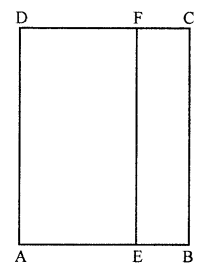Solution:
Side of the square ABCD = 24 cm
EF || BC || AB is drawn and AE = 15 cm
EB = 24 – 15 = 9 cm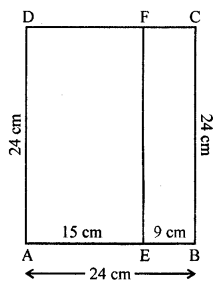(i) Now perimeter of AEFD = 2(15 + 24) cm = 2 × 39 = 78 cm
and perimeter of EBCF = 2(9 + 24) = 2 × 33 cm = 66 cm
Difference of perimeter = 78 – 66 = 12 cm
(ii) Now Area of AEFD = l × b = 15 × 24 = 360 sq. cm
and area of EBCF = 9 × 24 = 216 sq. cm
Difference = 360 – 216 = 144 sq. cm

Question 2.
Nagma runs around a rectangular park 180 m long and 120 m wide at the rate of 7.5 km/ hour. In how much time will she complete five rounds?
Solution:
Length of rectangular plot (l) = 180 m
and breadth (b) = 120 m
Perimeter = 2(l + b) = 2(180 + 120) m = 2 × 300 = 600 m
Distance travelled in 5 rounds = 600 × 5 = 3000 m = 3 km
Speed = 7.5 km/hrQuestion 3.
The area of a rectangular plot is 540 m2. if its length is 27 m, find its breadth and perimeter.
Solution:
Area of a rectangular plot = 540 m2
Length (l) = 27 mand perimeter = 2(l + b) = 2(27 + 20) m = 2 × 47 = 94 m

Question 4.
The perimeter of a rectangular field is 151 m. If its breadth is 32 m, find its length and area.
Solution:
Perimeter of a rectangular field = 151 m
Length =$$\frac { Perimeter }{ 2 }$$ – Breadth
= $$\frac { 151 }{ 2 }$$ – 32
= $$\frac { 87 }{ 2 }$$
= 43.5 m
and area = l × b = 43.5 × 32 m2 = 1392 m2

Question 5.
The area of a rectangular plot is 340 m2 and its breadth is 17 m. Find the cost of surrounding the plot with a fence at ₹ 5.70 per meter.
Solution:
Area of plot = 340 m2
and breadth (b) = 17 m
Length = $$\frac { A }{ b }$$ = $$\frac { 340 }{ 17 }$$ = 20 m
Perimeter = 2(l + b) = 2(20 + 17) = 2 × 37 = 74 m
Rate of fencing around it = ₹ 5.70 per m
Total cost = ₹5.70 × 74 = ₹ 421.80

Question 6.
The area of a square park is the same as that of a rectangular park. If the side of the square park is 60 m and the length of the rectangular park is 90 m, find the breadth of the rectangular park.
Solution:
Side of a square park = 60 m
Area = (Side)2 = 60 × 60 = 3600 m2
Area of rectangular park = 3600 m2
and length (l) = 90 m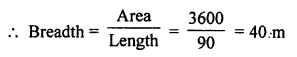Question 7.
A wire is in the shape of a rectangle. Its length is 40 cm and breadth is 22 cm. If the same wire is rebent in the shape of a square, what will be the measure of each side? Also, find which shape encloses more area and by how much?
Solution:
A wire in shape of a rectangle whose length (l) = 40 m
and breadth (b) = 22 m
Perimeter (Length) of wire = 2(l + b) = 2(40 + 22) cm = 2 × 62 cm = 124 cm
Perimeter of square wire = 124 cm
Then side = $$\frac { Perimeter }{ 4 }$$ = $$\frac { 124 }{ 4 }$$ = 31 m
Now area of rectangle = l × b = 40 × 22 = 880 cm2
and area of square = (Side)2 = (31)2 cm2 = 961 cm2
Difference in area = 961 – 880 = 81 cm2
Area of 81 cm2 is more of square shaped wire.

Question 8.
A door of breadth 1 m and height 2 m is fitted in a wall. The length of the wall is 4.5 m and the height is 3.6 m. Find the cost of whitewashing the wall, if the rate of whitewashing the wall is ₹ 20 per m2.
Solution:
Breadth of door = 1 m and height = 2 m
Area of door = l × b = 1 × 2 = 2 m2
Length of wall = 4.5 m and height = 3.6 m
Area = 4.5 × 3.6 m2 = 16.2 m2
Area of wall excluding area of door = 16.2 – 2 = 14.2 m2
Rate of white washing = ₹ 20 per m2
Total cost = 14.2 × 20 = ₹ 284

Question 9.
A rectangular park is 45 m long and 30 m wide. A path 2.5 m wide is constructed outside the park. Find the area of the path.
Solution:
Length of a rectangular park (l) = 45 m
and breadth (b) = 30 m
Width of path outside the park = 2.5 m
Outer length (L) = 45 + 2 × 2.5 m = 45 + 5 = 50 mand width (B) = 30 + 2 × 2.5 = 30 + 5 = 35 m
Area of park = L × B – l × b
= 50 × 35 – 45 × 30 m2
= 1750 – 1350
= 400 m2

Question 10.
A carpet of size 5 m × 2 m has 25 cm wide red border. The inner part of the carpet is blue in colour. Find the area of the blue portion. What is the ratio of the areas of red portion to blue portion?
Solution:
Length of blue carpet (l) = 5 m
Width of red border = 25 cm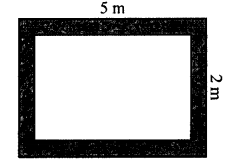Inner length = 5 – $$\frac { 2\times 25 }{ 100 }$$ = 5 – 0.5 = 4.5 m
and breadth = 2 – 0.5 = 1.5 m
Now area of carpet = 4.5 × 1.5 m2 = 6.75 m2
and area of border = 5 × 2 – 6.75 m2 = 10 – 6.75 = 3.25 m2
Now ratio between border and carpet (blue part) = 3.25 : 6.75 = 13 : 27

Question 11.
A verandah of width 2.25 m is constructed all along outside a room which is 5.5 m long and 4 m wide. Find:
(i) the area of the verandah.
(ii) the cost of cementing the floor of the verandah at the rate of ₹ 200 per m2.
Solution:
Width of a verandah = 2.25 m
Length of room (i) = 5.5 m
and breadth (b) = 4.0 m
Outer length (L) = 5.5 + 2 × 2.25 m = 5.5 + 4.5 = 10 m
and outer breadth = 4 + 4.5 = 8.5 m
(i) Area of verandah = Outer area – Inner area
= 10 × 8.5 – 5.5 × 4 m2
= 85 – 22 m2
= 63 m2
(ii) Rate of cementing the floor of verandah = ₹ 200 per m2
Total cost = ₹ 63 × 200 = ₹ 12600

Question 12.
Two crossroads, each of width 5 m, run at right angles through the centre of a rectangular park of length 70 m and breadth 45 m and parallel to its sides. Find the area of the roads. Also, find the cost of constructing the roads at the rate of ₹ 105 per m2.
Solution:
Length of rectangular park (l) = 70 m
and breadth (ft) = 45 m
Width of each road = 5 m(i) Area of roads = 70 × 5 + 45 × 5 – (5)2 m2
= 350 + 225 – 25 = 550 m2
(ii) Rate of constructing the roads = ₹105 per m2
Total cost = ₹ 105 × 550 = ₹ 57750

Question 13.
A rectangular room is 10 m long and 7.5 m wide. Find the cost of covering the floor with carpet 1.25 m wide at ₹ 250 per metre.
Solution:
Length of rectangular room (l) = 10 m
and breadth (b) = 7.5 m
Area of floor of the room = l × b = 10 × 7.5 = 75 m2
Width of carpet = 1.25 m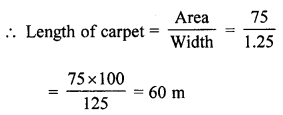Cost of 1 m carpet = ₹ 250
Total cost = ₹ 250 × 60 = ₹ 15000

Question 14.
Find the cost of flooring a room 6.5 m by 5 m with square tiles of side 25 cm at the rate of ₹ 9.40 per tile.
Solution:
Length of floor of a room (l) = 6.5 m
and breadth (b) = 5 m
Area = 6.5 × 5 m2 = 32.5 m2Cost of tile at the rate of ₹ 9.40 per tile = ₹ 9.40 × 520 = ₹ 4888

Question 15.
The floor of a room is in the shape of a square of side 4.8 m. The floor is to be covered with square tiles of perimeter 1.2 m. Find the cost of covering the floor if each tile costs ₹ 27.
Solution:
Side of square room = 4.8 m
Area = (4.8)2 m2 = 23.04 m2
Perimeter of one tile = 1.2 m
Side = $$\frac { 1.2 }{ 4 }$$ = 0.3 m
Area of one tile = (0.3)2 = 0.09 m2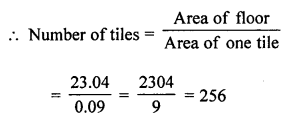Cost of one tile = ₹ 27
Total cost = 256 × ₹ 27 = ₹ 6912

Question 16.
A rectangular plot of land is 50 m wide. The cost of fencing the plot at the rate of ₹ 18 per metre is ₹ 4680. Find:
(i) the length of the plot.
(ii) the cost of leveling the plot at the rate of ₹ 7.6 per m2.
Solution:
Breadth of a plot = 50 m
Cost of fencing around it = ₹ 4680
Rate of fencing = ₹ 18 per m
Perimeter = $$\frac { 4680 }{ 18 }$$ = 260 m
Length =$$\frac { Perimeter }{ 2 }$$ – Breadth
= $$\frac { 260 }{ 2 }$$ – 50
= 130 – 50
= 80 m
(ii) Now area of plot = l × b = 80 × 50 m2 = 4000 m2
Rate of leveling the plot = ₹ 7.6 per m2
Total cost = ₹ 4000 × ₹ 7.6 = ₹ 30400